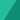### Yeri's Digital Note

August 24, 2016

You are doing some chmod now and then, and get lost..frequently. Then you’re definitely need to look at this. `r` = 4 `w` = 2 `x` = 1 `-` = 0   Example:

• `rwx r-- r-- (4+2+1) (4+0+0) (4+0+0) = 744`
• `rw- r-x r-x (4+2+0) (4+0+1) (4+0+1) = 655`
• the list goes on..

There you have it.Written by Yeri